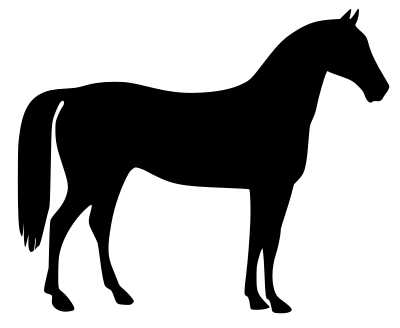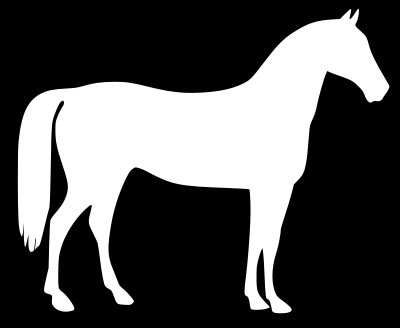# Invert image with Python, Pillow (Negative / positive inversion)

Posted: 2019-05-14 / Tags: Python, Pillow, Image Processing

In the `ImageOps` module of the Python image processing library Pillow (PIL), `invert()` for negative-positive inverting (inverting pixel values) of an image is provided.

`ImageChops` module has the same function.

See the following article for the installation and basic usage of Pillow (PIL).

See the following article for negative / positive inversion when reading an image as a NumPy array `ndarray` with OpenCV, etc.

## Sample code

Just load the image and call `invert()`.

``````from PIL import Image, ImageOps

im = Image.open('data/src/lena.jpg')
im_invert = ImageOps.invert(im)
im_invert.save('data/dst/lena_invert.jpg', quality=95)
``````If the transparent png is read by `open()`, `mode` is `RGBA` and it is not processed correctly, so convert to `RGB` by `convert()`.

most operators only work on L and RGB images.
ImageOps Module — Pillow (PIL Fork) 4.4.0.dev0 documentation

``````im = Image.open('data/src/horse.png').convert('RGB')
im_invert = ImageOps.invert(im)
im_invert.save('data/dst/horse_invert.png')
``````